# The Floods of the Nile¶

## History¶

The Nile river, in Egypt, played a crucial role in the development of the Egyptian civilization during antiquity. In particular its yearly summer floods would fertilize the arable land and allow the abundant production of food.

The authority of the Pharaoh was based on the belief that he was causing the flood at will. As a consequence, predicting the time and amplitude of the floods was key to his political power. This led to the recording of the level of the flood every year. One of the first known example of time-series recorded in history.

As we will see later in this notebook, modern study of the floods of the Nile led to the discovery of the so-called long range dependency effect. This effect occurs in many natural processes and reflect the fact that these processes have strong local correlation in time. This effect is also related to fractals and was also discovered by Mandelbrot when studying financial data.## The Data¶

The data we will play with is the monthly flow average at Dongola for years 1870 to 1984. Dongola is the station just upstream of Aswan. It is used instead of Aswan as after the High Aswan dam was build, the flow became regulated and doesn't actually reflect the natural variability in the flow.

The unit of the data is $m^3/s$.

But, let us first start pylab.

In :
%pylab inline

Populating the interactive namespace from numpy and matplotlib


We now import our data from a text file and reshape it into a vector. We create a time-indexed vector where the unit is one year and months are spaced by $\frac{1}{12}$. In the first few years, entries are missing, so we remove the data corresponding the these years.

In :
# Import data from a text file
fp = open("FloodsOfTheNile_data.txt", "rb")
FN = loadtxt(fp ,delimiter=",",skiprows=2)
fp.close()

# Linearize the matrix
amplitude = reshape(FN[:,1:-1], (-1,1))

# create time index, slices of 1/12 are for months
time = arange(FN[0,0],FN[-1,0]+1,1./12.)

# identify unavailable values
I = np.nonzero(amplitude >= 0)

# identify start of continuous values, start in january
s = (ceil(max(nonzero(amplitude < 0))/12)+1)*12

# compact all this
T = time[s:]
A = array([float(amplitude[i]) for i in range(int(s),len(amplitude))])


Now, let's take a first look at the data.

In :
figure()
plot(time[I], amplitude[I])
title('Floods of the Nile')
xlim(time, time[-1])
xlabel('Year')
ylabel('Flow [m$^3$/s]')
show()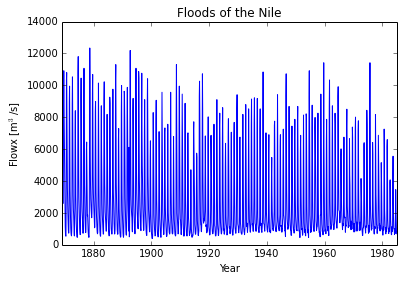This is quite dense. We also expect the curve to be very similar for every year. Let's try to overlap all the curves for every year in the dataset. We expect them to overlap quite tightly.

In :
figure()
plot(range(1,13), reshape(amplitude[s:], (-1,12)).T)
title('Yearly curves overlapped')
xlim((0.95, 12.05))
xticks(arange(1,13), ('jan','feb','mar','apr','may','jun','jul','aug','sep','oct','nov','dec'), rotation='vertical')
xlabel('Month')
ylabel('Flow [m$^3$/s]')
show()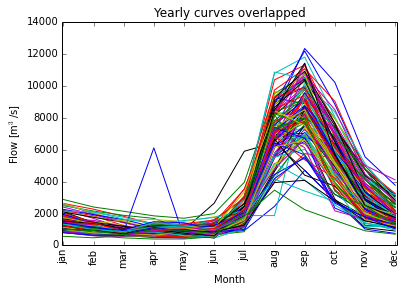To get further intuition about the variability of the flow during every month, we visualize the previous curve with boxplots, showing median, 0.25 quantiles and 0.75 quantiles, as well as extrema.

In :
figure()
boxplot(reshape(amplitude[s:], (-1,12)),1)
title('Yearly curves overlapped')
xlim((0.95, 12.05))
xticks(arange(1,13), ('jan','feb','mar','apr','may','jun','jul','aug','sep','oct','nov','dec'), rotation='vertical')
show()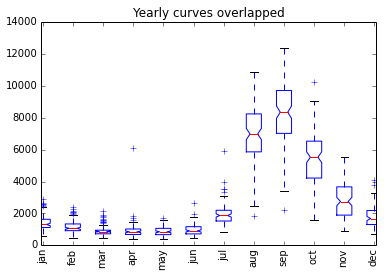From this, we make the following observation.

• During the dry months, the flow has a very low variablility.
• During the months corresponding to the flood (August to October), the flow has a very high variability.

From this simple observation, it would appear that the intensity of the flood is difficult to predict. As we will see, this is actually not the case.

## Long term dependence¶

A characteristic of many natural processes is a very long range dependence. The dependence here is how events are influencing each other with respect to their distance in time. In the case of the Nile, just like the Pharaoh of old time, we are interested in the amplitude of the yearly flood. From our previous study, we saw that the flood happens in September and we will limit our data to just this month.

In :
flood = A[8::12]
flood = [float(flood[i]) for i in range(len(flood))]
t = T[1::12]

plot(t, flood)
xlabel('Year')
ylabel('Flow [m$^3$/s]')
show()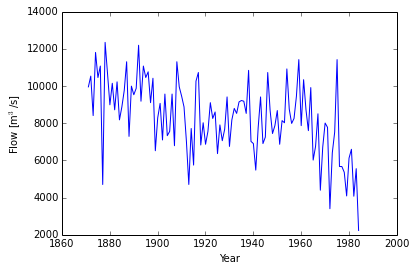The dependence of events of a time series can be quantified using the autocorrelation function (ACF). The value of the ACF is between 1, when two events are maximally correlated (they determine completely each other), and -1, when they are maximally anti-correlated (one is the opposite of the other). A value of 0 indicates that the two events are not correlated (the value of one has little or no relation with the other). The ACF measures this correlation as a function of how far apart in time events are.

For example, for a time series where successive events are independent, we expect the ACF to be 1 at zero (correlation of an event with itself), and zero everywhere else. In practice, we can only compute an approximation of the ACF, so instead of zero, we see a function clustered around zero.

For a process with long range dependence, the ACF follows a power law, i.e. the correlation between events decays as a power of their spacing in time:

$$ACF(t) = Ct^{-\alpha}.$$

As an example, we plot the empirical autocorrelation of the Nile flood data, and a time series of random independent data. One notices that the flood data ACF only decays slowly, while the ACF of random data is close to zero everywhere, except at 0.

A slow decaying ACF indicates a process that can be very well predicted. In other words, given the flood amplitude for several successive years, it is possible to fairly accurately guess what will be the next value of the process. In the case of the Nile, it means that several high floods are likely to be followed by a high flood, and vice versa. This was indeed the basis of the power of the Pharaoh.

In :
def acf(x):
R = zeros(size(x))
mu = mean(x)
sigma2 = std(x)**2
n = len(x)
for k in range(n):
R[k] = sum((x[0:n-k]-mu)*(x[k:]-mu))/(sigma2*(n-k))

return R

t = arange(0,len(flood)/2)
acf_flood = acf(flood)[:len(t)]
acf_rand = acf(rand(size(flood)))[:len(t)]

plot(t, acf_flood[:len(t)], 'b')
plot(t, acf_rand[:len(t)], 'r')
legend(('Flood data', 'Random'))
show()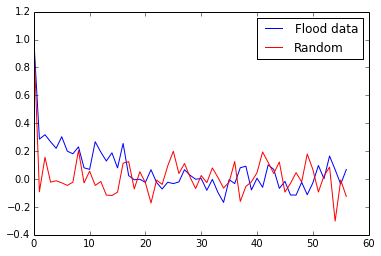Harold Edwin Hurst, a british hydrologist, is the father of modern Nile studies. He was in fact the first one to formalize the concept of long range dependency. He notably described the Hurst exponent, a characterization of the long range dependence of a process. The Hurst exponent is directly linked to the ACF in the following way. If the ACF is written as a power decay

$$ACF(t) = Ct^{-\alpha},$$

then, the Hurst exponent is

$$H = 1-\frac{\alpha}{2}.$$

The Hurst exponent can be estimated using a quantity called the rescaled range that we won't describe in further details here. For more information, one can consult wikipedia. We write a short routine to compute the rescaled range and the Hurst factor.

In :
# Compute the rescaled range for all partial time series of length n
def rescaled_range(X,n):

RS = 0

for i in range(int(len(X)-n)):

# compute the mean
m = mean(X[i:i+n])
# make zero mean
Y = X[i:i+n] - m
# partial cumulative sums
Z = cumsum(Y)
# difference max and min of this time-serie
R = max(Z) - min(Z)
# variance
S = sqrt(mean(Y**2))

RS += R/S

return RS/(len(X)-n)

# Routine to compute hurst factor
def hurst_factor(data):

# compute rescaled range for all size of partial sums
logmax = int(floor(log2(len(data))))
N = 2**arange(2,logmax+1)
RS = zeros(size(N))
for i in arange(logmax-1):
RS[i] = rescaled_range(data, N[i])

# compute the linear fit
M = reshape(concatenate((log(N.T), ones(N.shape).T), axis=0), (2,-1)).T
lf = dot(dot(inv(dot(M.T, M)), M.T), log(RS).T)

# Hurst parameter is the slope of the linear fit
return lf, lf, log(N), RS



While we expect the Hurst factor to be close to 0.5 for uncorrelated data, Hurst found a significant factor of 0.7 for the floods of the Nile. Let's see if we can find this from our data.

In :
# Compute Hurst factor
hf, lf, n, RS = hurst_factor(flood)

# plot empirical rescaled range and linear fit
plot(n, log(RS), 'b', n, lf+n*lf, 'r--')
xlim(n,n[-1])
xlabel('log(n)')
ylabel('log(R[n]/S[n])')
show()

print 'Hurst factor for the Floods of the Nile : %f' % lf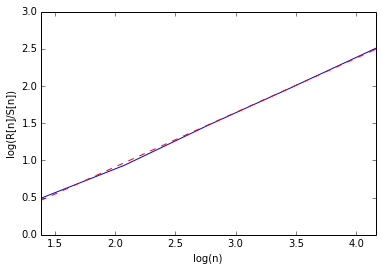Hurst factor for the Floods of the Nile : 0.732528


Fantastic. We can now check that this indeed follows the decay of the ACF.

In :
alpha = 2*(1-hf)
plot(t, acf_flood)
acf_decay = arange(0,len(acf_flood))**(-alpha)
plot(t, acf_decay,'r')
show()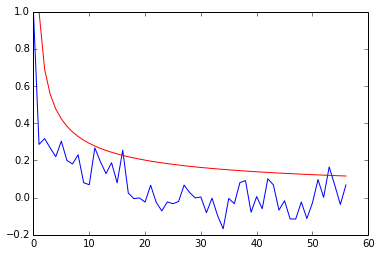And finally, we check for random uncorrelated data.

In :
hfr, lfr, nr, RSr = hurst_factor(randn(1000))

# plot empirical rescaled range
plot(nr, log(RSr),'b', nr, lfr*nr+lfr, 'r--')
xlim((nr,nr[-1]))
ylabel('log(R/S)')
show()

print 'Hurst factor for random data : %f' % lfr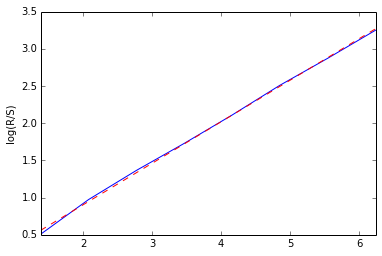Hurst factor for random data : 0.558146


## Study the discrete Fourier transform¶

As an added bonus, the flow of the Nile is naturally a quasi periodic signal (but for the variable flood level). This gives us a chance to observe the Fourier transform of such signals. In particular, we know that for a perfectly periodic signal, the Fourier coefficients will be zero but at integer frequencies. Let's observe what happens here using the DFT. Since we have many periods, this should be a reasonnable approximation to compute the continuous Fourier series:

$$X_k = \sum_{n=0}^{N-1} x_n e^{j2\pi \frac{nk}{N}}.$$

Rather than the dry linear index of the vector, we would like to know what the frequency corresponds to. Our time unit is in year, and our sampling period is one month or

$$T_s = \frac{1}{12}.$$

Let in addition $N$ be the signal length and $T$ the signal duration (in years). We have the following relations:

$$T = N\,T_s,$$$$F_s = \frac{1}{T_s}.$$

Thus, our sampling period in the frequency domain is

$$\Delta F = \frac{F_s}{N} = \frac{1}{T}.$$

Since our signal is real, the DFT is conjugate symmetric and we only need to keep $N/2+1$ first samples.

In :
N = len(A)
Ts = 1./12.
Ttot = N*Ts

# Compute the DFT
F = fft.fft(A.T).T

# since the signal is real, we can just keep one half of the spectrum
F = F[:N/2+1]

# construct the corresponding frequency vector
freq = arange(0, N/2+1)/Ttot

# display the magnitude of the spectrum
figure()
plot(freq, np.abs(F))
xlabel('1/year')
ylabel('|dft(A)|')
xlim((-0.1,6.1))
show()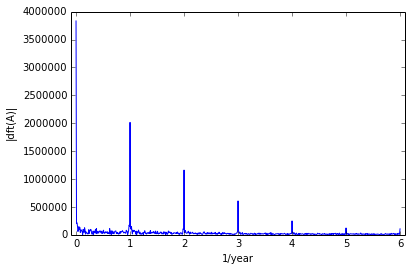First, we see the expected behavior, i.e. integer frequencies are dominating since our signal is quasi-periodic. However, we observe that low frequencies (between 0 and 1) are not quite close to zero, as a close-up reveals. Here, we first zero the integer coefficient and then plot the residue signal.

In :
zero_range = range(0, len(F), int(Ttot))
new_F = F
new_F[zero_range] = 0
figure()
plot(freq, abs(new_F))
xlabel('1/year')
ylabel('|dft(A)|')
xlim((-0.1,6.1))
show()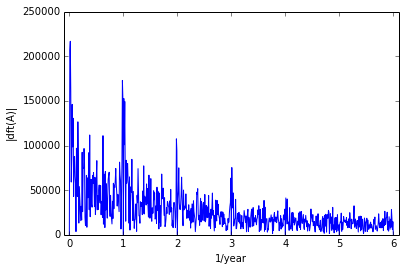We observe some relatively strong components in the very low frequency domain, which might be explained by the long range dependency of the data.